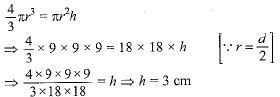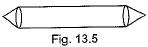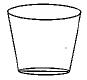Courses

# MCQ : Surface Area And Volumes - 2

## 10 Questions MCQ Test Mathematics (Maths) Class 10 | MCQ : Surface Area And Volumes - 2

Description
This mock test of MCQ : Surface Area And Volumes - 2 for Class 10 helps you for every Class 10 entrance exam. This contains 10 Multiple Choice Questions for Class 10 MCQ : Surface Area And Volumes - 2 (mcq) to study with solutions a complete question bank. The solved questions answers in this MCQ : Surface Area And Volumes - 2 quiz give you a good mix of easy questions and tough questions. Class 10 students definitely take this MCQ : Surface Area And Volumes - 2 exercise for a better result in the exam. You can find other MCQ : Surface Area And Volumes - 2 extra questions, long questions & short questions for Class 10 on EduRev as well by searching above.
QUESTION: 1

Solution:
QUESTION: 2

Solution:
QUESTION: 3

### A sphere of diameter 18 cm is dropped into a cylindrical vessel of diameter 36 cm, partly filled with water. If the sphere is completely submerged, then the water level rises (in cm) by

Solution:

Volume of sphere
= Volume of water in cylindrical vessel∴ Water level rises by 3 cm

QUESTION: 4

The shape of a gilli, in the gilli-danda game figure is a combination ofSolution:
QUESTION: 5

A right circular cylinder of radius r cm and height h cm (h > 2r) just encloses a sphere of diameter

Solution:
QUESTION: 6

A hollow cube of internal edge 22 cm is filled with spherical marbles of diameter 0.5 cm and it is assumed tha 1/8 space of the cube remains unfilled. Then the number of marbles that the cube can accommodate is

Solution:
QUESTION: 7

During conversion of a solid from one shape to another, the volume of the new shape will

Solution:
QUESTION: 8

The shape of a glass (tumbler) (see Fig.) is usually in the form ofSolution:
QUESTION: 9

A mason constructs a wall of dimensions 270 cm x 300 cm x 350 cm with the bricks each of size 22.5 cm x 11.25 cm x 8.75 cm and it is assumed that 1/8 space is covered by the mortar.
Then the number of bricks used to construct the wall is

Solution:
QUESTION: 10

The radii of the top and bottom of a bucket of slant height 45 cm are 28 cm and 7 cm respectively. The curved surface area of the bucket is

Solution: